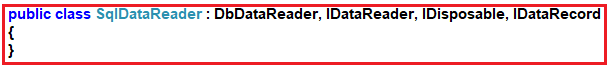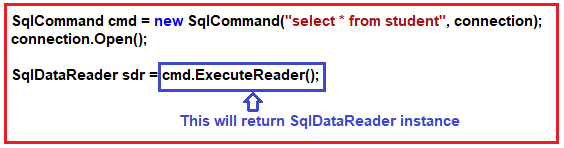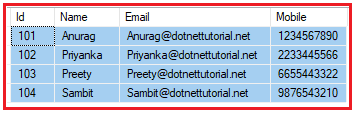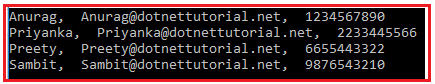1. What is SqlDataReader Class and its need?
2. How to create an instance of the SqlDataReader class.

The ADO.NET SqlDataReader class in C# is used to read data from SQL Server database in the most efficient manner. It reads data in forward-only stream. It means, once it read a record, it will then read the next record, there is no way to go back and read the previous record.

The SqlDataReader is connection oriented. It means it requires an open or active connection to the data source while reading the data. The data is available as long as the connection with database exists

SqlDataReader is read-only. It means it is also not possible to change the data using SqlDataReader. You also need to open and close the connection explicitly.

If you look at the following image, you will see that, this class is inherits from DbDataReader class and implements IDisposable interface.The SqlDataReader class provides the following properties.

1. Connection: It gets the System.Data.SqlClient.SqlConnection associated with the System.Data.SqlClient.SqlDataReader.
2. Depth: It gets a value that indicates the depth of nesting for the current row.
3. FieldCount: It gets the number of columns in the current row.
4. HasRows: It gets a value that indicates whether the System.Data.SqlClient.SqlDataReader contains one or more rows.
5. IsClosed: It retrieves a Boolean value that indicates whether the specified System.Data.SqlClient.SqlDataReader instance has been closed.
6. RecordsAffected: It gets the number of rows changed, inserted, or deleted by execution of the Transact-SQL statement.
7. VisibleFieldCount: It gets the number of fields in the System.Data.SqlClient.SqlDataReader that are not hidden.
8. Item[String]: It gets the value of the specified column in its native format given the column name.
9. Item[Int32]: It gets the value of the specified column in its native format given the column ordinal.

The SqlDataReader class provides the following methods.

1. Close(): It closes the SqlDataReader object.
2. GetBoolean(int i): It gets the value of the specified column as a Boolean. Here, the parameter i is the zero-based column ordinal.
3. GetByte(int i): It gets the value of the specified column as a byte. Here, the parameter i is the zero-based column ordinal.
4. GetChar(int i): It gets the value of the specified column as a single character. Here, the parameter i is the zero-based column ordinal.
5. GetDateTime(int i): It gets the value of the specified column as a System.DateTime object. Here, the parameter i is the zero-based column ordinal.
6. GetDecimal(int i): It gets the value of the specified column as a System.Decimal object. Here, the parameter i is the zero-based column ordinal.
7. GetDouble(int i): It gets the value of the specified column as a double-precision floating point number. Here, the parameter i is the zero-based column ordinal.
8. GetFloat(int i): It gets the value of the specified column as a single-precision floating point number. Here, the parameter i is the zero-based column ordinal.
9. GetName(int i): It gets the name of the specified column. Here, the parameter i is the zero-based column ordinal.
10. GetSchemaTable(): It returns a System.Data.DataTable that describes the column metadata of the System.Data.SqlClient.SqlDataReader
11. GetValue(int i): It gets the value of the specified column in its native format. Here, the parameter i is the zero-based column ordinal.
12. GetValues(object[] values): It Populates an array of objects with the column values of the current row. Here, the parameter values is an array of System.Object into which to copy the attribute columns.
13. NextResult(): It advances the data reader to the next result, when reading the results of batch Transact-SQL statements.
14. Read(): It Advances the System.Data.SqlClient.SqlDataReader to the next record and returns true if there are more rows; otherwise false.

You can not create the instance of SqlDataReader using the new keyword. Then the question is how we get or create the instance of SqlDataReader class. In order to create the instance of SqlDataReader class, what you  need to do is, call the ExecuteReader method of the SqlCommand object which will return an instance of SqlDataReader class as shown in the below image.We are going to use the following student table to understand the SqlDataReader object in C#.Please use below SQL script to create a database called StudentDB, a table called Student with the required test data.

```CREATE DATABASE StudentDB;
GO

USE StudentDB;
GO

CREATE TABLE Student(
Id INT PRIMARY KEY,
Name VARCHAR(100),
Email VARCHAR(50),
Mobile VARCHAR(50)
)
GO

INSERT INTO Student VALUES (101, 'Anurag', 'Anurag@dotnettutorial.net', '1234567890')
INSERT INTO Student VALUES (102, 'Priyanka', 'Priyanka@dotnettutorial.net', '2233445566')
INSERT INTO Student VALUES (103, 'Preety', 'Preety@dotnettutorial.net', '6655443322')
INSERT INTO Student VALUES (104, 'Sambit', 'Sambit@dotnettutorial.net', '9876543210')
GO```
##### Example: Using SqlDataReader in C#

We need to fetch all the data from the student table and need to display in the console using SqlDataReader. The following code exactly does the same thing. In the below example, we use the Read() method of SqlDataReader object to loop through the items of SqlDataReader object. The Read method returns true as long as there is rows to read from the SqlDataReader object. If there is no more rows to read, then this method will simply return false.

```using System;
using System.Data.SqlClient;
{
class Program
{
static void Main(string[] args)
{
try
{
string ConString = "data source=.; database=StudentDB; integrated security=SSPI";
using (SqlConnection connection = new SqlConnection(ConString))
{
// Creating the command object
SqlCommand cmd = new SqlCommand("select * from student", connection);

// Opening Connection
connection.Open();

// Executing the SQL query

//Looping through each record
{
Console.WriteLine(sdr["Name"] + ",  " + sdr["Email"] + ",  " + sdr["Mobile"]);
}
}
}
catch (Exception e)
{
Console.WriteLine("OOPs, something went wrong.\n" + e);
}